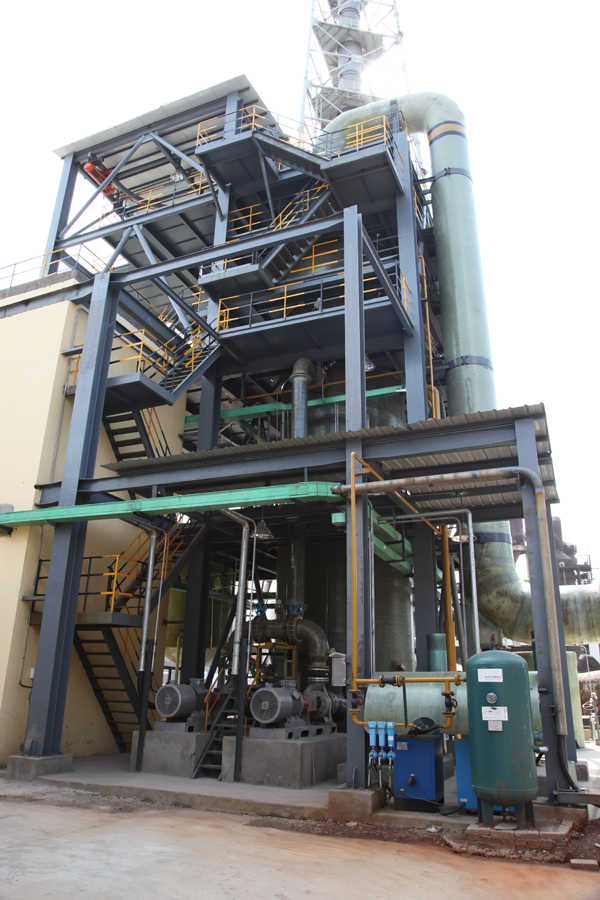（1）吸收过程

SO2   +  H2O  =   H2SO3

H2SO3   +  ZnO  +  1.5H2O =   ZnSO3·2.5H2

（2）

ZnSO3·2.5H2O +  1/2O2    =   ZnSO4   +  2.5H2O

（3）

ZnSO3·2.5H2O   +  H2SO4   =   ZnSO  +  SO2↑ + 3.5H2O